# Angles And Polygons Mathematics 10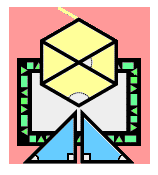## Keith Seidler and Romesh Kachroo

1993
S 105.10

### INTRODUCTION

To meet a need for resources for the new Math 10 curriculum, the Saskatchewan Teachers' Federation, in cooperation with Saskatchewan Education, Training and Employment, initiated the development of teacher-prepared unit plans.
A group of teachers who had piloted the course in 1992-93 were invited to a two and a half day workshop in August, 1993 at the STF. The teachers worked alone or in pairs to develop a plan for a section of the course.
Jim Beamer, University of Saskatchewan, and Lyle Markowski, Saskatchewan Education, Training and Employment, acted as resource persons for the workshop.

TEACHER NOTE:

Throughout the sample units, the following symbols are used to refer to the Common Essential Learnings:
```C 	Communication
CCT 	Critical and Creative Thinking
IL 	Independent Learning
N 	Numeracy
PSVS 	Personal and Social Values and Skills
TL 	Technological Literacy
```

Section One
Section Two: Properties of Polygons
Section Three
Section Four:The Pythagorean Theory
Section Five: Trigonometric Ratios

## Foundational Objectives:

1. To identify and apply common properties of triangles, special quadrilaterals, and n-gons. (10 05 01). Supported by learning objectives 1, 2, 3, 4, 8, 9, and 10.
2. To apply the special quadrilaterals to real world situations. (10 05 02). Supported by learning objectives 6 and 7.
3. To develop an understanding of Pythagoras' Theorem, the primary trigonometric ratios, and their applications. (10 05 03). Supported by learning objectives 11 to 17.

## Objectives:

• To define and illustrate terms related to the study of angles.
• To solve diagrammatic and word problems involving these angles.

## Background:

Most terms should be familiar to students from previous mathematics courses. Some explanation may be required for regular polygons.

2 periods

## Instructional Methods or Activities:

Note: Activity 2 could be done before or after Activity 1 or left out. Activity 3 is intended as an adaptive dimension.

1. Students may use the following activity sheet to review, define, and illustrate angular terms. Following this, the students may be assigned suitable questions involving these terms. (IL, N, CCT) Examples from Geometry - Jurgensen 1985 edition could include:

Page Numbers and suitable questions:

• page 13 classroom exercises 1-38
• page 14 written exercises 1-38
• page 31 classroom exercises 1-18
• page 32 written exercises 1-32 challenge 35-38

2. Students may be broken into groups of 3 or 4 in which they discuss occupations involving a knowledge of angles (see pages 120-121 of mathematical curriculum guide). Presentations may be as suggested or a guest speaker may be invited into class to discuss how his/her occupation involves angles or to demonstrate to students any special equipment that occupation may use to measure angles. (PSVS, C, CCT, N)

Adaptive Dimension Students may be broken into groups to develop board games which combine the above objectives. Such games may be designed as a treasure hunt or as saving your best friend from certain doom. In this game, students must solve word problems which involve angles - the solution to the problem tells them the angular direction they should move to advance. The distance of the move may be given or found be solving algebraic equations. This game could be adapted to include different types of angular measurement - e.g. N30E or headings of 120 degrees. (CCT, TL, N, PSVS)

## Assessment:

• informal questioning
• anecdotal records

## ACTIVITY SHEET #1 - ANGLES

(Use straight edge for diagrams)
```Term		 	Illustration			 Definition
```

* angle

* acute angle

* right angle

* obtuse angle

* straight angle

* reflex angle

```Term 		Illustration 			Definition
```

* complementary angles

* supplementary angles

* vertical angles

* congruent angles

* central angle of a regular polygon

## Concept:

Properties of polygons

## Objectives:

(3, 4, 5, 6 of the Curriculum Guide)
• To define and illustrate terms related to polygons.
• To classify quadrilaterals and polygons.
• To construct types of parallelograms.
• To state and apply properties of parallelograms.

3 periods

## Instructional Methods or Activities:

Note: The teacher may decide to use some or all of the following depending upon students.

1. The students may be grouped into 3 or 4 for the following concept mapping activity. Each group will require a large sheet of bristol board and an envelope containing two or three of each of the following polygons of varying sizes or shapes: triangles (scalene, isosceles, equilateral, acute, right, obtuse); quadrilaterals (with no sides congruent both convex and non-convex, trapezoids, isosceles trapezoids, parallelograms, rectangles, rhombuses and squares); pentagons, hexagons, and octagons (at least one of each of these should be regular).

Students would be required to set up a classification system for all above shapes, using the properties of the shapes (see A-2.03 curriculum guide instructional notes page 122). When completed, each group should be requested to explain their classification system. Geometric properties may be summarized on board - consistencies and inconsistencies of each system may be discussed. (CCT, C ,PSVS,)

2. Students need to study the properties of quadrilaterals and more specifically parallelograms. Students may complete a chart such as the following or page 171 - Jurgensen. Encourage students to draw sketches to experiment with each characteristic, or use MIRA to test some. (IL, TL)
Alternately use the chart on page 171, Jurgensen 1985 as an assessment chart.
3. The teacher may wish to use the following Venn diagram to help explain the properties of quadrilaterals from general to specific.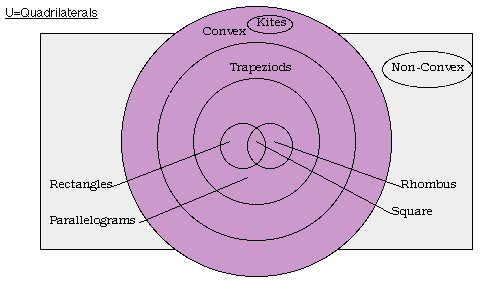4. Student discussions could be used to explore methods of informally constructing parallelograms, rectangles, rhombuses, and squares. Paper folding and MIRA are options as suggested in the curriculum guide, page 122, as is the mechanical method using straight edge, rulers, and set squares. If formal constructions were covered previously (see page 118 of curriculum guide), then this may also be used. Students are encouraged to come up with other methods. (C, PSVS, CCT)

1. Students may be encouraged to search for examples of polygons in nature. For example, the hexagons of honeycombs, pentagons on shells of turtles, polygons on body of giraffes, etc. Polygons are also used in construction, machinery, jewelry, etc. Presentations may be made in the form of posters where diagrams may be hand-drawn or pictures from magazines or as oral presentations of applications of polygons in specific occupations. Alternatively, students may wish to create picture-art in which all elements of the diagram are made from polygons or compass. Students may be encouraged to find out how to construct kaleidoscopes. See media house catalogue for available videos.

## Assessment:

• On-going student activity
• Activity or assessment sheet following
• Homework
• Presentation Concept mapping
• group assessment
• presentation assessment

### ASSESSMENT WORKSHEET FOR QUIZ/ASSIGNMENT PROPERTIES OF POLYGONS WORKSHEET

(Use straight edge for diagrams)

```Term 		Illustration 		Properties and/or Characteristics
```

* polygon

* regular polygon

* convex polygon

* non-convex polygon

* triangle

* acute triangle

* right triangle

* scalene triangle

* obtuse triangle

```					Definition and/or
Term		Illustration	Properties and/or Characteristics

* isosceles triangle

* equilateral triangle

* parallelogram

* rectangle

* rhombus

* square

* trapezoid

* isosceles polygon

SECTION THREE

Objectives: (7, 8, 9, 10 from Curriculum Guide)

To determine the sum of the measures of the interior and exterior
angles of a convex polygon of n sides.
To determine the measure of a central angle in a regular n-gon.
To determine the measures of the interior and exterior angles of regular
n-gons.
To determine the number of diagonals in a polygon of n sides from each
vertex.

Background:
Students should be able to draw and/or identify central angles, exterior
(external) angles, and interior angles of regular polygons.

Equipment needed:

Protractor
straight edge

Time:
2 or 3 periods

Instructional Method and/or Activities:

Note: The following exercises may be preceded by a discussion
on polygons in general and extended to regular polygons. The teacher may
show which properties are similar to both groups.

Students may work in groups of 2 to complete exercises 1 and 2 (attached),
and prepare a report to the class. Alternatively, the teacher may have
groups meet and debate their results before presentations are made to the
larger body. (PSVS, C, CCT, N)
Exercise 3 may then be completed in groups or as the teacher feels
appropriate.
Students may require some help to fill in the row dealing with n-gons.
(Exercise 3) With guided discussion, some students may develop the sum
of interior angle formula. (IL, CCT, N, C)
Students will need more practice in questions related to above. Geometry
Jurgensen 1985, pages 80-84. (IL, N)

Assessment:

Presentation
Group Assessment

Evaluation:
The teacher may choose to administer a quiz or test at this point of this
unit.

Exercise 1:
Draw the central angle -C and one external angle -E for each of the following
regular polygons. Measure these and complete the table below. Write a short
conclusion at the bottom of the page.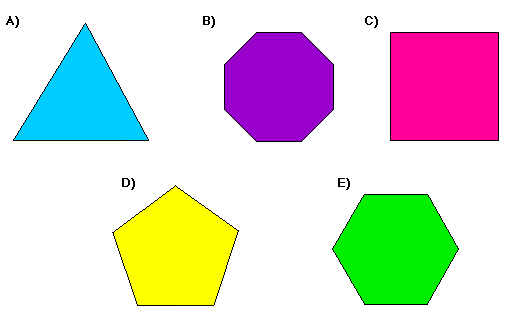Conclusion:

m "greater than"C		m  "greater than"E

A
B
C
D
E

Exercise 2:
Measure one interior angle (a) and one exterior angle (b) of each of the
following regular polygons.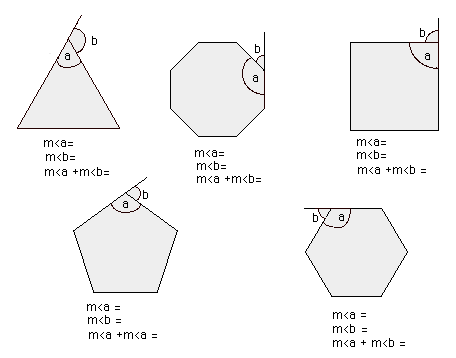Since all the interior angles of a regular polygon are equal and all the
exterior angles of a regular polygon are equals.

Find the sum of interior angles of:
A)
B)
C)
D)
E)

Find the sum of exterior angles of:
A)
B)
C)
D)
E)

Exercise 3:
The following information can be arranged into a table format.
Figure out the following information when looking at polygons with 3, 4,
5, 5, 7, 8, 9, 10 sides.

Number of Diagonals (from same vertex)
Number of Triangles
Sum of Interior Angles
measurment  of int. <'s of regular polygon
measurement  of ext. <'s of regular polygon
m. of central Angle
sum of all the exterior angles

SECTION FOUR:  The Pythorgorean Theory

Concept:
The Pythagorean Theorem

Objectives:
(11, 12, 13 from Curriculum Guide)

To calculate to two decimal places the length of a missing side
of a right triangle using the Pythagorean Theorem.
To solve word problems using the Pythagorean Theorem.
To determine of a triangle is a right triangle by using the converse
of the Pythagorean Theorem.

Background:
Knowledge of terminology of sides of right triangle; stress hypotenuse
as the longest side, opposite the right angle; knowledge of solving simple
equations, the meaning of the square root and use of a calculator to determine
square roots.

Time:
2 or 3 periods
Instructional Methods and/or Activities:
Note: The teacher may want to bring in the historical perspective
of the development of the Pythagorean Theorem. (Video "Donald in Mathmagic
Land" by Disney may be fun)

The students may require some practice in determining the square root
of teacher specified numbers using the calculator. (TL, N, IL)
The teacher may introduce the Pythagorean Theorem giving several examples
on the board by altering the position of the right angle in the diagram.
The teacher may have students estimate the value of the sides before determining
the values using calculators. (CC, IL, N) (See sample pages on Pythagorean
Theorem attached). Additional questions may be found in:

Addison-Wesley, Math 10 (1987) - pages 380-391
Principles and Process 9(1988) - pages 106-107
Geometry Jurgensen (1985) - pages 252-259
selective Math Is 4 - pages 37-42
Principles and Process 10 - pages 56-57

Evaluation:

Quiz or Test

Pythagorean Theorem:
The Pythagorean theorem states:
In a right triangle the square of the hypotenuse equals the sum of the
squares of the other two sides.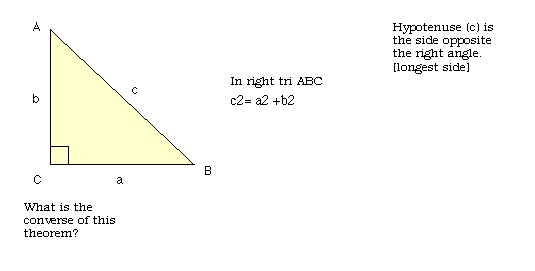Examples: (On Board)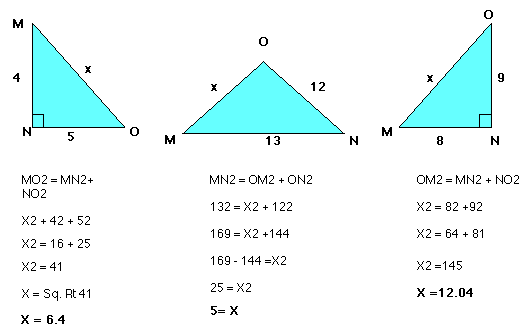Exercises:
(Diagrams are not drawn to scale)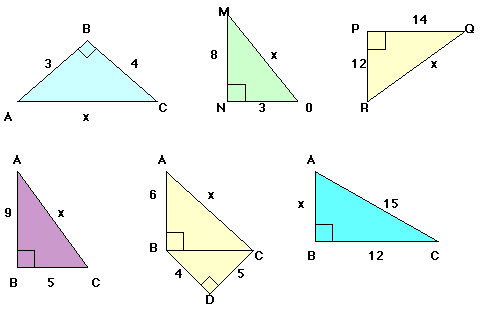b) Which of the following are not right triangles? Give a reason.
(Note: Triangles may not be exactly as shown)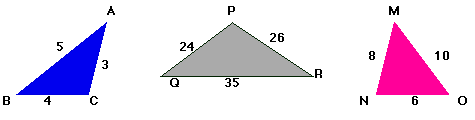SECTION FIVE:  Trigonometric Ratios

Angles and Polygons Math 10
Concept:
Trigonometric Ratios

Objectives:
(14, 15, 16, 17 from Curriculum Guide)

To determine the value of the three primary trigonometric ratios
using a calculator or tables.
To determine the measure of an angle given the value of one trigonometric
ratio of the angle by using the calculator or tables.
To calculate the measure of an angle or the length of a side of a right
triangle using the tangent, sine, and cosine ratios.
To solve word problems that involve trigonometric ratios using a
calculator.

Background:
Knowledge of right triangle and Pythagorean Theorem

Time:
3 periods
Instructional Methods and/or Activities:

The students may require practice in labeling the right angle, triangles
in terms of hypotenuse, side adjacent and side opposite with reference
to one of the two acute angles. (IL, CCT)
Example: *ABC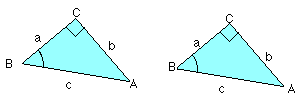In both diagrams the hypotenuse remains the same, however, the sides
adjacent and opposite are interchanged when the reference angle is changed.
Students should become familiar with determining the sine, cosine,
and tangent values by use of calculator (or tables if necessary). (TL,
N, IL)
A more formal introduction of the trigonometric ratios may be used,
in terms of hypotenuse, sides adjacent and opposite. A sample introduction
on trigonometric ratios is included. Examples should lead into word problems
and applications of these trigonometric ratios. Angles of evaluation and
depression should be included. (CCT, C, N, IL, PSVS, TL)
Sample exercises may be found:

Holt Math 10 (1987), pages 332-340

Holt, Teachers Resource Manual (1987) - Master 13-1 and 13-2

Math Is 4, pages 332-340

Principles and Process 10 (1992), pages 462-480

The teacher may use the concepts of similar triangles when introducing
trigonometric ratios.

Evaluation: (Quiz on Trigonometric Ratios)
Comprehensive Test on unit of Angles and Polygons may be given.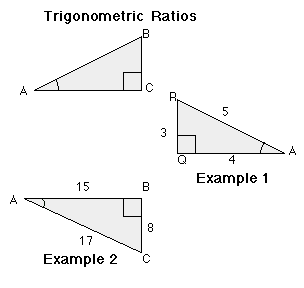Trigonometric Ratios:

Sin function Sin A = opposite A
hypotenuse

Cos function Cos A = side adjacent A
hypotenuse

Tan function Tan A = side opposite

Example 1:
In *PQR let us find Sin P, Cos P, Tan P

Sin P = opposite A = 3
hypotenuse 5

Cos P = side adjacent A = 4
hypotenuse 5

Tan P = side opposite = 3

Example 2:
In *ABC

Sin C =

Cos C =

Tan C =

Unit Angles and Polygons References:

Geometry, Jurgensen, et all, 1985, Houghton Mifflin.
Geometry, Clemens, et al, 1984, Addison-Wesley Publishing Co.
Principles and Process 9, Ebos, et al, 1988, Nelson.
Principles and Process 10, Ebos, et al, 1992, Nelson.
Holt Math 10, Bye, Dale, et al, 1987, Holt, Rinehart and Winston.
Holt Math 10, Teachers Resource Manual, 1987, Holt, Rinehart and
Winston.
Math Is 4, Ebos & Tuck, 1979, Nelson.

This unit comes from the The Stewart Resources Centre which
provides
library resources and teacher-prepared materials for teachers in Saskatchewan.
To borrow materials or obtain a free catalogue listing unit and lesson
plans contact :

Stewart Resources Centre,
2317 Arlington Avenue,
phone 306-373-1660; fax  306-374-1122,
e-mail  src@stf.sk.ca.
http://www.stf.sk.ca/

Go to Math Central